# Kendall tau metric

Jump to: navigation, search

Kendall tau

The non-parametric correlation coefficient (or measure of association) known as Kendall's tau was first discussed by G.T. Fechner and others about 1900, and was rediscovered (independently) by M.G. Kendall in 1938 [a3], [a4]. In modern use, the term "correlation" refers to a measure of a linear relationship between variates (such as the Pearson product-moment correlation coefficient), while "measure of association" refers to a measure of a monotone relationship between variates (such as Kendall's tau and the Spearman rho metric). For a historical review of Kendall's tau and related coefficients, see [a5].

Underlying the definition of Kendall's tau is the notion of concordance. If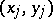and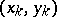are two elements of a samplefrom a bivariate population, one says thatandare concordant ifand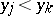or if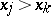and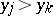(i.e., if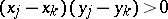); and discordant if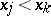andor ifand(i.e., if). There are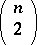distinct pairs of observations in the sample, and each pair (barring ties) is either concordant or discordant. Denoting bythe numberof concordant pairs minus the numberof discordant pairs, Kendall's tau for the sample is defined asWhen ties exist in the data, the following adjusted formula is used: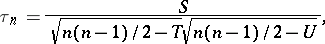where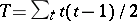forthe number ofobservations that are tied at a given rank, and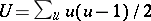forthe number ofobservations that are tied at a given rank. For details on the use of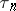in hypotheses testing, and for large-sample theory, see [a2].

Note thatis equal to the probability of concordance minus the probability of discordance for a pair of observationsandchosen randomly from the sample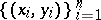. The population versionof Kendall's tau is defined similarly for random variablesand(cf. also Random variable). Let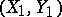and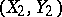be independent random vectors with the same distribution as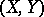. Then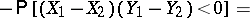Sinceis the Pearson product-moment correlation coefficient of the random variablesand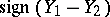,is sometimes called the difference sign correlation coefficient.

Whenandare continuous,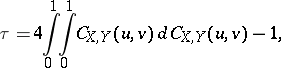where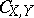is the copula ofand. Consequently,is invariant under strictly increasing transformations ofand, a propertyshares with Spearman's rho, but not with the Pearson product-moment correlation coefficient. For a survey of copulas and their relationship with measures of association, see [a6].

Besides Kendall's tau, there are other measures of association based on the notion of concordance, one of which is Blomqvist's coefficient [a1]. Letdenote a sample from a continuous bivariate population, and let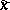anddenote sample medians (cf. also Median (in statistics)). Divide the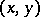-plane into four quadrants with the linesand; and let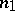be the number of sample points belonging to the first or third quadrants, and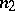the number of points belonging to the second or fourth quadrants. If the sample sizeis even, the calculation ofandis evident. Ifis odd, then one or two of the sample points fall on the lines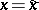and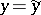. In the first case one ignores the point; in the second case one assigns one point to the quadrant touched by both points and ignores the other. Then Blomqvist'sis defined asFor details on the use ofin hypothesis testing, and for large-sample theory, see [a1].

The population parameter estimated by, denoted by, is defined analogously to Kendall's tau (cf. Kendall tau metric). Denoting by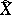and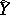the population medians ofand, then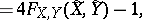wheredenotes the joint distribution function ofand. Sincedepends only on the value of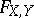at the point whose coordinates are the population medians ofand, it is sometimes called the medial correlation coefficient. Whenandare continuous,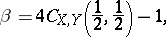whereagain denotes the copula ofand. Thus, like, is invariant under strictly increasing transformations ofand.